Documentation

Case Study for a Credit Scorecard Analysis

This example shows how to create a creditscorecard object, bin data, display, and plot binned data information. This example also shows how to fit a logistic regression model, obtain a score for the scorecard model, and determine the probabilities of default and validate the credit scorecard model using three different metrics.

Step 1. Create a creditscorecard object.

Use the CreditCardData.mat file to load the data (using a dataset from Refaat 2011). If your data contains many predictors, you can first use screenpredictors from Risk Management Toolbox™ to pare down a potentially large set of predictors to a subset that is most predictive of the credit scorecard response variable. You can then use this subset of predictors when creating the creditscorecard object.

When creating a creditscorecard object, by default, 'ResponseVar' is set to the last column in the data ('status' in this example) and the 'GoodLabel' to the response value with the highest count (0 in this example). The syntax for creditscorecard indicates that 'CustID' is the 'IDVar' to remove from the list of predictors. Also, while not demonstrated in this example, when creating a creditscorecard object using creditscorecard, you can use the optional name-value pair argument 'WeightsVar' to specify observation (sample) weights or 'BinMissingData' to bin missing data.

sc = creditscorecard(data,'IDVar','CustID')
sc =
creditscorecard with properties:

GoodLabel: 0
ResponseVar: 'status'
WeightsVar: ''
VarNames: {1x11 cell}
NumericPredictors: {1x6 cell}
CategoricalPredictors: {'ResStatus'  'EmpStatus'  'OtherCC'}
BinMissingData: 0
IDVar: 'CustID'
PredictorVars: {1x9 cell}
Data: [1200x11 table]

Perform some initial data exploration. Inquire about predictor statistics for the categorical variable 'ResStatus' and plot the bin information for 'ResStatus'.

bininfo(sc,'ResStatus')
ans=4×6 table
Bin          Good    Bad     Odds        WOE       InfoValue
______________    ____    ___    ______    _________    _________

{'Home Owner'}    365     177    2.0621     0.019329    0.0001682
{'Tenant'    }    307     167    1.8383    -0.095564    0.0036638
{'Other'     }    131      53    2.4717      0.20049    0.0059418
{'Totals'    }    803     397    2.0227          NaN    0.0097738

plotbins(sc,'ResStatus')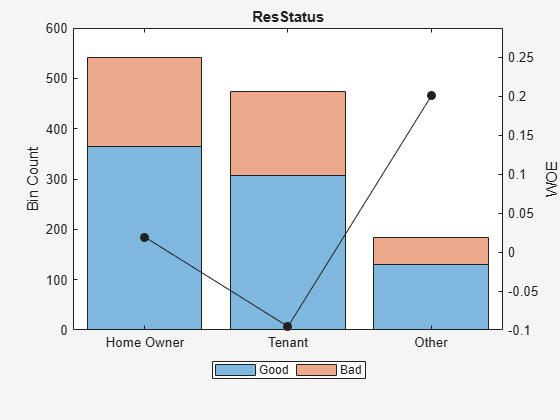This bin information contains the frequencies of “Good” and “Bad,” and bin statistics. Avoid having bins with frequencies of zero because they lead to infinite or undefined (NaN) statistics. Use the modifybins or autobinning functions to bin the data accordingly.

For numeric data, a common first step is "fine classing." This means binning the data into several bins, defined with a regular grid. To illustrate this point, use the predictor 'CustIncome'.

cp = 20000:5000:60000;

sc = modifybins(sc,'CustIncome','CutPoints',cp);

bininfo(sc,'CustIncome')
ans=11×6 table
Bin           Good    Bad     Odds         WOE       InfoValue
_________________    ____    ___    _______    _________    __________

{'[-Inf,20000)' }      3       5        0.6      -1.2152      0.010765
{'[20000,25000)'}     23      16     1.4375     -0.34151     0.0039819
{'[25000,30000)'}     38      47    0.80851     -0.91698      0.065166
{'[30000,35000)'}    131      75     1.7467     -0.14671      0.003782
{'[35000,40000)'}    193      98     1.9694    -0.026696    0.00017359
{'[40000,45000)'}    173      76     2.2763      0.11814     0.0028361
{'[45000,50000)'}    131      47     2.7872      0.32063      0.014348
{'[50000,55000)'}     82      24     3.4167      0.52425      0.021842
{'[55000,60000)'}     21       8      2.625      0.26066     0.0015642
{'[60000,Inf]'  }      8       1          8        1.375      0.010235
{'Totals'       }    803     397     2.0227          NaN       0.13469

plotbins(sc,'CustIncome')Step 2a. Automatically bin the data.

Use the autobinning function to perform automatic binning for every predictor variable, using the default 'Monotone' algorithm with default algorithm options.

sc = autobinning(sc);

After the automatic binning step, every predictor bin must be reviewed using the bininfo and plotbins functions and fine-tuned. A monotonic, ideally linear trend in the Weight of Evidence (WOE) is desirable for credit scorecards because this translates into linear points for a given predictor. The WOE trends can be visualized using plotbins.

plotbins(sc,sc.PredictorVars)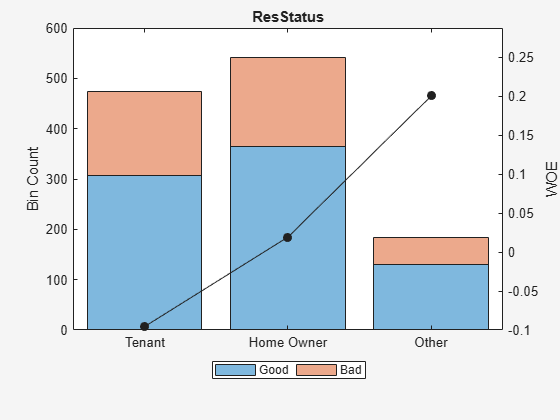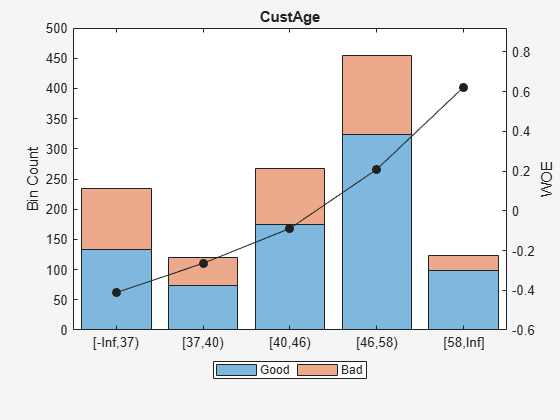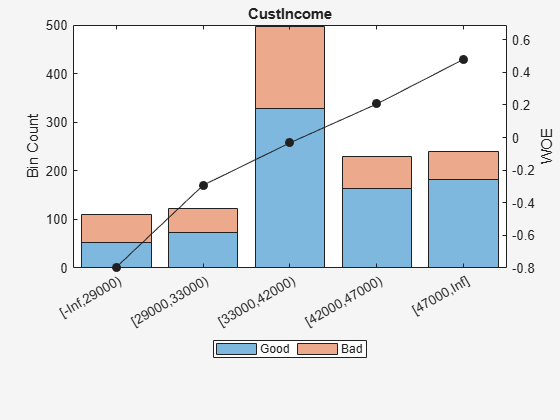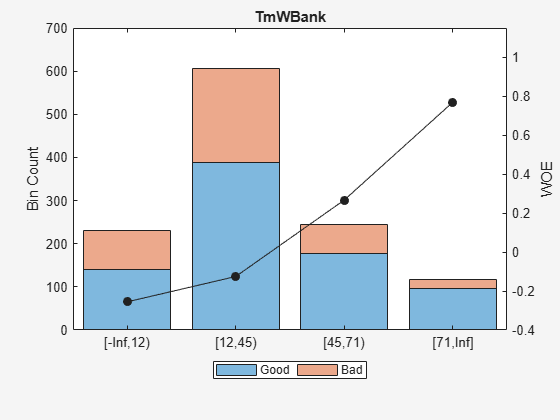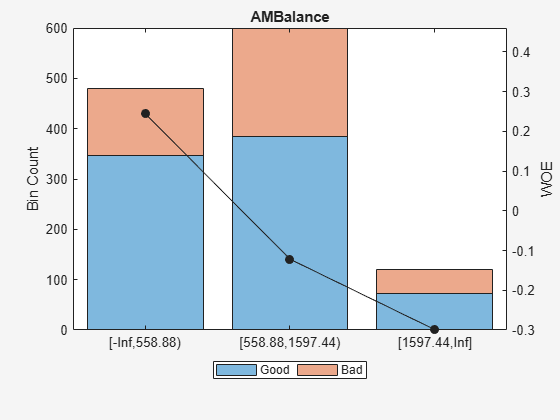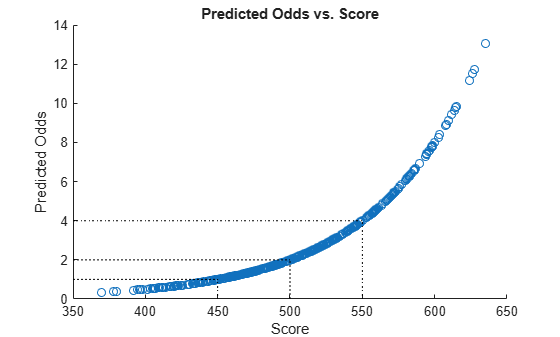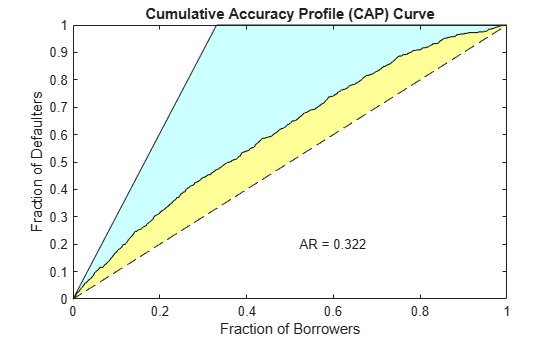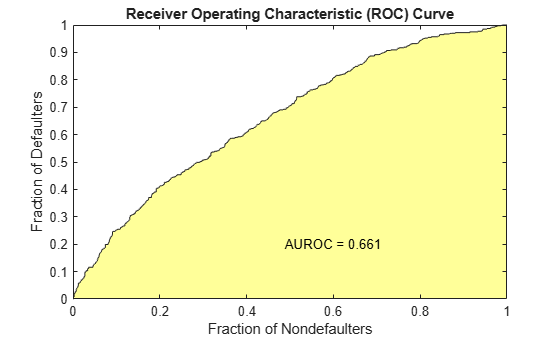Unlike the initial plot of 'ResStatus' when the scorecard was created, the new plot for 'ResStatus' shows an increasing WOE trend. This is because the autobinning function, by default, sorts the order of the categories by increasing odds.

These plots show that the 'Monotone' algorithm does a good job finding monotone WOE trends for this dataset. To complete the binning process, it is necessary to make only a few manual adjustments for some predictors using the modifybins function.

Step 2b. Fine-tune the bins using manual binning.

Common steps to manually modify bins are:

• Use the bininfo function with two output arguments where the second argument contains binning rules.

• Manually modify the binning rules using the second output argument from bininfo.

• Set the updated binning rules with modifybins and then use plotbins or bininfo to review the updated bins.

For example, based on the plot for 'CustAge' in Step 2a, bins number 1 and 2 have similar WOE's as do bins number 5 and 6. To merge these bins using the steps outlined above:

[bi,cp] = bininfo(sc,'CustAge');
cp([1 5]) = []; % To merge bins 1 and 2, and bins 5 and 6
sc = modifybins(sc,'CustAge','CutPoints',cp);
plotbins(sc,'CustAge')For 'CustIncome', based on the plot above, it is best to merge bins 3, 4 and 5 because they have similar WOE's. To merge these bins:

[bi,cp] = bininfo(sc,'CustIncome');
cp([3 4]) = [];
sc = modifybins(sc,'CustIncome','CutPoints',cp);
plotbins(sc,'CustIncome')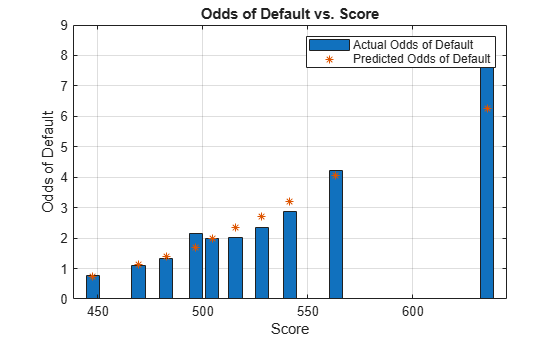For 'TmWBank', based on the plot above, it is best to merge bins 2 and 3 because they have similar WOE's. To merge these bins:

[bi,cp] = bininfo(sc,'TmWBank');
cp(2) = [];
sc = modifybins(sc,'TmWBank','CutPoints',cp);
plotbins(sc,'TmWBank')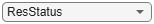For 'AMBalance', based on the plot above, it is best to merge bins 2 and 3 because they have similar WOE's. To merge these bins:

[bi,cp] = bininfo(sc,'AMBalance');
cp(2) = [];
sc = modifybins(sc,'AMBalance','CutPoints',cp);
plotbins(sc,'AMBalance')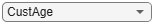Now that the binning fine-tuning is completed, the bins for all predictors have close-to-linear WOE trends.

Step 3. Fit a logistic regression model.

The fitmodel function fits a logistic regression model to the WOE data. fitmodel internally bins the training data, transforms it into WOE values, maps the response variable so that 'Good' is 1, and fits a linear logistic regression model. By default, fitmodel uses a stepwise procedure to determine which predictors should be in the model.

sc = fitmodel(sc);
1. Adding CustIncome, Deviance = 1490.8954, Chi2Stat = 32.545914, PValue = 1.1640961e-08
2. Adding TmWBank, Deviance = 1467.3249, Chi2Stat = 23.570535, PValue = 1.2041739e-06
3. Adding AMBalance, Deviance = 1455.858, Chi2Stat = 11.466846, PValue = 0.00070848829
4. Adding EmpStatus, Deviance = 1447.6148, Chi2Stat = 8.2432677, PValue = 0.0040903428
5. Adding CustAge, Deviance = 1442.06, Chi2Stat = 5.5547849, PValue = 0.018430237
6. Adding ResStatus, Deviance = 1437.9435, Chi2Stat = 4.1164321, PValue = 0.042468555
7. Adding OtherCC, Deviance = 1433.7372, Chi2Stat = 4.2063597, PValue = 0.040272676

Generalized linear regression model:
status ~ [Linear formula with 8 terms in 7 predictors]
Distribution = Binomial

Estimated Coefficients:
Estimate      SE       tStat       pValue
________    _______    ______    __________

(Intercept)     0.7024       0.064    10.975    5.0407e-28
CustAge        0.61562     0.24783    2.4841      0.012988
ResStatus       1.3776     0.65266    2.1107      0.034799
EmpStatus      0.88592     0.29296     3.024     0.0024946
CustIncome     0.69836     0.21715     3.216     0.0013001
TmWBank          1.106     0.23266    4.7538    1.9958e-06
OtherCC         1.0933     0.52911    2.0662      0.038806
AMBalance       1.0437     0.32292    3.2322     0.0012285

1200 observations, 1192 error degrees of freedom
Dispersion: 1
Chi^2-statistic vs. constant model: 89.7, p-value = 1.42e-16

Step 4. Review and format scorecard points.

After fitting the logistic model, by default the points are unscaled and come directly from the combination of WOE values and model coefficients. The displaypoints function summarizes the scorecard points.

p1 = displaypoints(sc);
disp(p1)
Predictors              Bin              Points
______________    ____________________    _________

{'CustAge'   }    {'[-Inf,37)'       }     -0.15314
{'CustAge'   }    {'[37,40)'         }    -0.062247
{'CustAge'   }    {'[40,46)'         }     0.045763
{'CustAge'   }    {'[46,58)'         }      0.22888
{'CustAge'   }    {'[58,Inf]'        }      0.48354
{'CustAge'   }    {'<missing>'       }          NaN
{'ResStatus' }    {'Tenant'          }    -0.031302
{'ResStatus' }    {'Home Owner'      }      0.12697
{'ResStatus' }    {'Other'           }      0.37652
{'ResStatus' }    {'<missing>'       }          NaN
{'EmpStatus' }    {'Unknown'         }    -0.076369
{'EmpStatus' }    {'Employed'        }      0.31456
{'EmpStatus' }    {'<missing>'       }          NaN
{'CustIncome'}    {'[-Inf,29000)'    }     -0.45455
{'CustIncome'}    {'[29000,33000)'   }      -0.1037
{'CustIncome'}    {'[33000,42000)'   }     0.077768
{'CustIncome'}    {'[42000,47000)'   }      0.24406
{'CustIncome'}    {'[47000,Inf]'     }      0.43536
{'CustIncome'}    {'<missing>'       }          NaN
{'TmWBank'   }    {'[-Inf,12)'       }     -0.18221
{'TmWBank'   }    {'[12,45)'         }    -0.038279
{'TmWBank'   }    {'[45,71)'         }      0.39569
{'TmWBank'   }    {'[71,Inf]'        }      0.95074
{'TmWBank'   }    {'<missing>'       }          NaN
{'OtherCC'   }    {'No'              }       -0.193
{'OtherCC'   }    {'Yes'             }      0.15868
{'OtherCC'   }    {'<missing>'       }          NaN
{'AMBalance' }    {'[-Inf,558.88)'   }       0.3552
{'AMBalance' }    {'[558.88,1597.44)'}    -0.026797
{'AMBalance' }    {'[1597.44,Inf]'   }     -0.21168
{'AMBalance' }    {'<missing>'       }          NaN

This is a good time to modify the bin labels, if this is something of interest for cosmetic reasons. To do so, use modifybins to change the bin labels.

sc = modifybins(sc,'CustAge','BinLabels',...
{'Up to 36' '37 to 39' '40 to 45' '46 to 57' '58 and up'});

sc = modifybins(sc,'CustIncome','BinLabels',...
{'Up to 28999' '29000 to 32999' '33000 to 41999' '42000 to 46999' '47000 and up'});

sc = modifybins(sc,'TmWBank','BinLabels',...
{'Up to 11' '12 to 44' '45 to 70' '71 and up'});

sc = modifybins(sc,'AMBalance','BinLabels',...
{'Up to 558.87' '558.88 to 1597.43' '1597.44 and up'});

p1 = displaypoints(sc);
disp(p1)
Predictors               Bin              Points
______________    _____________________    _________

{'CustAge'   }    {'Up to 36'         }     -0.15314
{'CustAge'   }    {'37 to 39'         }    -0.062247
{'CustAge'   }    {'40 to 45'         }     0.045763
{'CustAge'   }    {'46 to 57'         }      0.22888
{'CustAge'   }    {'58 and up'        }      0.48354
{'CustAge'   }    {'<missing>'        }          NaN
{'ResStatus' }    {'Tenant'           }    -0.031302
{'ResStatus' }    {'Home Owner'       }      0.12697
{'ResStatus' }    {'Other'            }      0.37652
{'ResStatus' }    {'<missing>'        }          NaN
{'EmpStatus' }    {'Unknown'          }    -0.076369
{'EmpStatus' }    {'Employed'         }      0.31456
{'EmpStatus' }    {'<missing>'        }          NaN
{'CustIncome'}    {'Up to 28999'      }     -0.45455
{'CustIncome'}    {'29000 to 32999'   }      -0.1037
{'CustIncome'}    {'33000 to 41999'   }     0.077768
{'CustIncome'}    {'42000 to 46999'   }      0.24406
{'CustIncome'}    {'47000 and up'     }      0.43536
{'CustIncome'}    {'<missing>'        }          NaN
{'TmWBank'   }    {'Up to 11'         }     -0.18221
{'TmWBank'   }    {'12 to 44'         }    -0.038279
{'TmWBank'   }    {'45 to 70'         }      0.39569
{'TmWBank'   }    {'71 and up'        }      0.95074
{'TmWBank'   }    {'<missing>'        }          NaN
{'OtherCC'   }    {'No'               }       -0.193
{'OtherCC'   }    {'Yes'              }      0.15868
{'OtherCC'   }    {'<missing>'        }          NaN
{'AMBalance' }    {'Up to 558.87'     }       0.3552
{'AMBalance' }    {'558.88 to 1597.43'}    -0.026797
{'AMBalance' }    {'1597.44 and up'   }     -0.21168
{'AMBalance' }    {'<missing>'        }          NaN

Points are usually scaled and also often rounded. To do this, use the formatpoints function. For example, you can set a target level of points corresponding to a target odds level and also set the required points-to-double-the-odds (PDO).

TargetPoints = 500;
TargetOdds = 2;
PDO = 50; % Points to double the odds

sc = formatpoints(sc,'PointsOddsAndPDO',[TargetPoints TargetOdds PDO]);
p2 = displaypoints(sc);
disp(p2)
Predictors               Bin             Points
______________    _____________________    ______

{'CustAge'   }    {'Up to 36'         }    53.239
{'CustAge'   }    {'37 to 39'         }    59.796
{'CustAge'   }    {'40 to 45'         }    67.587
{'CustAge'   }    {'46 to 57'         }    80.796
{'CustAge'   }    {'58 and up'        }    99.166
{'CustAge'   }    {'<missing>'        }       NaN
{'ResStatus' }    {'Tenant'           }    62.028
{'ResStatus' }    {'Home Owner'       }    73.445
{'ResStatus' }    {'Other'            }    91.446
{'ResStatus' }    {'<missing>'        }       NaN
{'EmpStatus' }    {'Unknown'          }    58.777
{'EmpStatus' }    {'Employed'         }    86.976
{'EmpStatus' }    {'<missing>'        }       NaN
{'CustIncome'}    {'Up to 28999'      }    31.497
{'CustIncome'}    {'29000 to 32999'   }    56.805
{'CustIncome'}    {'33000 to 41999'   }    69.896
{'CustIncome'}    {'42000 to 46999'   }    81.891
{'CustIncome'}    {'47000 and up'     }     95.69
{'CustIncome'}    {'<missing>'        }       NaN
{'TmWBank'   }    {'Up to 11'         }    51.142
{'TmWBank'   }    {'12 to 44'         }    61.524
{'TmWBank'   }    {'45 to 70'         }    92.829
{'TmWBank'   }    {'71 and up'        }    132.87
{'TmWBank'   }    {'<missing>'        }       NaN
{'OtherCC'   }    {'No'               }    50.364
{'OtherCC'   }    {'Yes'              }    75.732
{'OtherCC'   }    {'<missing>'        }       NaN
{'AMBalance' }    {'Up to 558.87'     }    89.908
{'AMBalance' }    {'558.88 to 1597.43'}    62.353
{'AMBalance' }    {'1597.44 and up'   }    49.016
{'AMBalance' }    {'<missing>'        }       NaN

Step 5. Score the data.

The score function computes the scores for the training data. An optional data input can also be passed to score, for example, validation data. The points per predictor for each customer are provided as an optional output.

[Scores,Points] = score(sc);
disp(Scores(1:10))
528.2044
554.8861
505.2406
564.0717
554.8861
586.1904
441.8755
515.8125
524.4553
508.3169
disp(Points(1:10,:))
CustAge    ResStatus    EmpStatus    CustIncome    TmWBank    OtherCC    AMBalance
_______    _________    _________    __________    _______    _______    _________

80.796      62.028       58.777         95.69      92.829     75.732      62.353
99.166      73.445       86.976         95.69      61.524     75.732      62.353
80.796      62.028       86.976        69.896      92.829     50.364      62.353
80.796      73.445       86.976         95.69      61.524     75.732      89.908
99.166      73.445       86.976         95.69      61.524     75.732      62.353
99.166      73.445       86.976         95.69      92.829     75.732      62.353
53.239      73.445       58.777        56.805      61.524     75.732      62.353
80.796      91.446       86.976         95.69      61.524     50.364      49.016
80.796      62.028       58.777         95.69      61.524     75.732      89.908
80.796      73.445       58.777         95.69      61.524     75.732      62.353

Step 6. Calculate the probability of default.

To calculate the probability of default, use the probdefault function.

pd = probdefault(sc);

Define the probability of being “Good” and plot the predicted odds versus the formatted scores. Visually analyze that the target points and target odds match and that the points-to-double-the-odds (PDO) relationship holds.

ProbGood = 1-pd;
PredictedOdds = ProbGood./pd;

figure
scatter(Scores,PredictedOdds)
title('Predicted Odds vs. Score')
xlabel('Score')
ylabel('Predicted Odds')

hold on

xLimits = xlim;
yLimits = ylim;

% Target points and odds
plot([TargetPoints TargetPoints],[yLimits(1) TargetOdds],'k:')
plot([xLimits(1) TargetPoints],[TargetOdds TargetOdds],'k:')

% Target points plus PDO
plot([TargetPoints+PDO TargetPoints+PDO],[yLimits(1) 2*TargetOdds],'k:')
plot([xLimits(1) TargetPoints+PDO],[2*TargetOdds 2*TargetOdds],'k:')

% Target points minus PDO
plot([TargetPoints-PDO TargetPoints-PDO],[yLimits(1) TargetOdds/2],'k:')
plot([xLimits(1) TargetPoints-PDO],[TargetOdds/2 TargetOdds/2],'k:')

hold off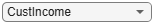Step 7. Validate the credit scorecard model using the CAP, ROC, and Kolmogorov-Smirnov statistic

The creditscorecard class supports three validation methods, the Cumulative Accuracy Profile (CAP), the Receiver Operating Characteristic (ROC), and the Kolmogorov-Smirnov (K-S) statistic. For more information on CAP, ROC, and KS, see Cumulative Accuracy Profile (CAP), Receiver Operating Characteristic (ROC), and Kolmogorov-Smirnov statistic (KS).

[Stats,T] = validatemodel(sc,'Plot',{'CAP','ROC','KS'});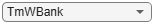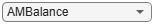disp(Stats)
Measure              Value
________________________    _______

{'Accuracy Ratio'      }    0.32225
{'Area under ROC curve'}    0.66113
{'KS statistic'        }    0.22324
{'KS score'            }     499.18
disp(T(1:15,:))
Scores    ProbDefault    TrueBads    FalseBads    TrueGoods    FalseGoods    Sensitivity    FalseAlarm      PctObs
______    ___________    ________    _________    _________    __________    ___________    __________    __________

369.4       0.7535          0           1           802          397                 0     0.0012453     0.00083333
377.86      0.73107          1           1           802          396         0.0025189     0.0012453      0.0016667
379.78       0.7258          2           1           802          395         0.0050378     0.0012453         0.0025
391.81      0.69139          3           1           802          394         0.0075567     0.0012453      0.0033333
394.77      0.68259          3           2           801          394         0.0075567     0.0024907      0.0041667
395.78      0.67954          4           2           801          393          0.010076     0.0024907          0.005
396.95      0.67598          5           2           801          392          0.012594     0.0024907      0.0058333
398.37      0.67167          6           2           801          391          0.015113     0.0024907      0.0066667
401.26      0.66276          7           2           801          390          0.017632     0.0024907         0.0075
403.23      0.65664          8           2           801          389          0.020151     0.0024907      0.0083333
405.09      0.65081          8           3           800          389          0.020151      0.003736      0.0091667
405.15      0.65062         11           5           798          386          0.027708     0.0062267       0.013333
405.37      0.64991         11           6           797          386          0.027708      0.007472       0.014167
406.18      0.64735         12           6           797          385          0.030227      0.007472          0.015
407.14      0.64433         13           6           797          384          0.032746      0.007472       0.015833